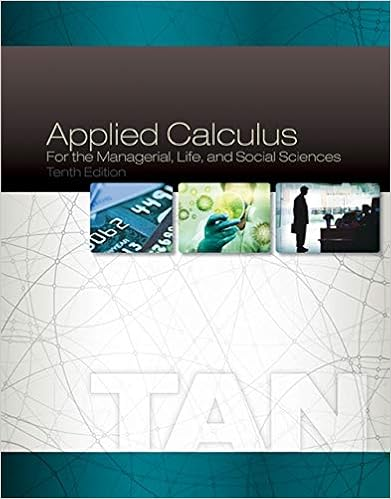# Equate coefficients of same degree variable x on the

• Notes
• 87
• 100% (1) 1 out of 1 people found this document helpful

This preview shows page 76 - 80 out of 87 pages.

##### We have textbook solutions for you!
The document you are viewing contains questions related to this textbook.The document you are viewing contains questions related to this textbook.
Chapter 8 / Exercise 47
Applied Calculus for the Managerial, Life, and Social Sciences
TanExpert Verified
Equate coefficients of same degree variable x on the left with those on the right sie of the equation * : x 2 : 1 = A + B + C (1) x : 4 = 2A + 4B (2) k : -1 = -3A + 3B C (3) (1) + (3) : 0 = -2A + 4B (4) (2) (4) : 4 = 4A A = 1 Substitute A = 1 in (2): 4 = 2(1) + 4B B = 1/2 Solving for C, use (1): 1 = 1 + 1/2 + C C = -1/2 Hence,   2 0 2 0 2 0 2 0 2 3 1 1 3 1 1 1 4 dx x C dx x B dx x A dx x x x x x 2 0 2 0 2 0 dx 3 x 2 / 1 dx 1 x 2 / 1 dx 1 x 1
##### We have textbook solutions for you!
The document you are viewing contains questions related to this textbook.The document you are viewing contains questions related to this textbook.
Chapter 8 / Exercise 47
Applied Calculus for the Managerial, Life, and Social Sciences
TanExpert Verified
  2 0 2 3 1 1 1 4 dx x x x x x 2 0 3 x ln 2 1 1 x ln 2 1 1 x ln 3 0 ln 3 2 ln 2 1 1 0 ln 1 2 ln 2 1 1 0 ln 1 2 ln 3 ln 5 ln 3 ln 2 2 1 1 ln 1 ln 2 1 1 ln 3 ln 3 ln 5 ln 2 1 3 ln 2 1 5 ln 2 1 3 ln 5 27 ln 2 1 5 ln 3 ln 3 2 1 CASE 2: REPEATED LINEAR FACTOR OF THE DENOMINATOR If the linear factor n b ax appears as the denominator of the rational function, for each repeated linear factor of the denominator, there corresponds a series of partial fractions, n 3 2 b ax N ... b ax C b ax B b ax A , (to make each partial fraction independent of the other) where A, B, C, …, N are constants to be determined. The degree n of the repeated linear factor gives the number of partial fractions in a series. Thus, dx b ax N ... dx b ax C dx b ax B dx b ax A dx ) x ( g ) x ( f n 3 2 Example 18.3 Evaluate dy y y 4 y 4 3 y 2 3 Solution: dy 1 y 2 y 3 y dy 1 y 4 y 4 y 3 y dy y y 4 y 4 3 y 2 2 2 3 2 2 2 1 y 2 y 1 y 2 C 1 y 2 B y A 1 y 2 y 3 y     y C 1 y 2 y B 1 y 2 A 3 y 2
Cy y y 2 B 1 y 4 y 4 A 3 y 2 2 Solving for A, B, and C: y 2 : 0 = 4A + 2B (1) y : 1 = 4A + B + C (2) k: 3 = A (3) Substitute A = 3 in (1): 0 = 12A + B B = 12 From (2) : 1 = 12 12 + C C = 1 dy 1 y 2 C dy 1 y 2 B dy y A dy 1 y 2 y 3 y 2 2 dy 1 y 2 1 dy 1 y 2 12 dy y 3 2 dy 2 1 y 2 2 1 dy 2 1 y 2 1 2 1 12 dy y 1 3 2 C 1 1 y 2 2 1 1 y 2 ln 6 y ln 3 1 C 1 y 2 1 1 y 2 ln 12 y ln 6 2 1 C 1 y 2 1 1 y 2 y ln 6 2 1 2 C 1 y 2 1 1 y 2 y ln 2 1 12 6 Example 18.4 Evaluate dx x x 3 x 3 x 1 x 2 3 4 5 2
Solution: dx 1 x x 1 x dx 1 x 3 x 3 x x 1 x dx x x
•••